The advection schemes known as centered second order, centered fourth order, first order upwind and upwind biased third order are known as linear advection schemes because the coefficient for interpolation of the advected tracer are linear and a function only of the flow, not the tracer field it self. We discuss these first since they are most commonly used in the field and most familiar.

## 2.17.1.1. Centered second order advection-diffusion¶

The basic discretization, centered second order, is the default. It is designed to be consistent with the continuity equation to facilitate conservation properties analogous to the continuum. However, centered second order advection is notoriously noisy and must be used in conjunction with some finite amount of diffusion to produce a sensible solution.

(2.148)${\cal A}_c \Delta r_f h_c G_{adv}^\tau = \delta_i F_x + \delta_j F_y + \delta_k F_r$

where the area integrated fluxes are given by:

\begin{split}\begin{aligned} F_x & = & U \overline{ \tau }^i \\ F_y & = & V \overline{ \tau }^j \\ F_r & = & W \overline{ \tau }^k\end{aligned}\end{split}

The quantities $$U$$, $$V$$ and $$W$$ are volume fluxes. defined as:

\begin{split}\begin{aligned} U & = & \Delta y_g \Delta r_f h_w u \\ V & = & \Delta x_g \Delta r_f h_s v \\ W & = & {\cal A}_c w\end{aligned}\end{split}

For non-divergent flow, this discretization can be shown to conserve the tracer both locally and globally and to globally conserve tracer variance, $$\tau^2$$. The proof is given in Adcroft (1995) [Adc95] and Adcroft et al. (1997) [AHM97] .

$$F_x$$ : uT ( argument )
$$U$$ : uTrans ( argument )
$$\tau$$ : tracer ( argument )

$$F_y$$ : vT ( argument )
$$V$$ : vTrans ( argument )
$$\tau$$ : tracer ( argument )

$$F_r$$ : wT ( argument )
$$W$$ : rTrans ( argument )
$$\tau$$ : tracer ( argument )

## 2.17.1.2. Third order upwind bias advection¶

Upwind biased third order advection offers a relatively good compromise between accuracy and smoothness. It is not a “positive” scheme meaning false extrema are permitted but the amplitude of such are significantly reduced over the centered second order method.

The third order upwind fluxes are discretized:

\begin{split}\begin{aligned} F_x & = & U \overline{\tau - \frac{1}{6} \delta_{ii} \tau}^i + \frac{1}{2} |U| \delta_i \frac{1}{6} \delta_{ii} \tau \\ F_y & = & V \overline{\tau - \frac{1}{6} \delta_{ii} \tau}^j + \frac{1}{2} |V| \delta_j \frac{1}{6} \delta_{jj} \tau \\ F_r & = & W \overline{\tau - \frac{1}{6} \delta_{ii} \tau}^k + \frac{1}{2} |W| \delta_k \frac{1}{6} \delta_{kk} \tau \end{aligned}\end{split}

At boundaries, $$\delta_{\hat{n}} \tau$$ is set to zero allowing $$\delta_{nn}$$ to be evaluated. We are currently examine the accuracy of this boundary condition and the effect on the solution.

$$F_x$$ : uT ( argument )
$$U$$ : uTrans ( argument )
$$\tau$$ : tracer ( argument )

$$F_y$$ : vT ( argument )
$$V$$ : vTrans ( argument )
$$\tau$$ : tracer ( argument )

$$F_r$$ : wT ( argument )
$$W$$ : rTrans ( argument )
$$\tau$$ : tracer ( argument )

## 2.17.1.3. Centered fourth order advection¶

Centered fourth order advection is formally the most accurate scheme we have implemented and can be used to great effect in high resolution simulations where dynamical scales are well resolved. However, the scheme is noisy, like the centered second order method, and so must be used with some finite amount of diffusion. Bi-harmonic is recommended since it is more scale selective and less likely to diffuse away the well resolved gradient the fourth order scheme worked so hard to create.

The centered fourth order fluxes are discretized:

\begin{split}\begin{aligned} F_x & = & U \overline{\tau - \frac{1}{6} \delta_{ii} \tau}^i \\ F_y & = & V \overline{\tau - \frac{1}{6} \delta_{ii} \tau}^j \\ F_r & = & W \overline{\tau - \frac{1}{6} \delta_{ii} \tau}^k\end{aligned}\end{split}

As for the third order scheme, the best discretization near boundaries is under investigation but currently $$\delta_i \tau=0$$ on a boundary.

$$F_x$$ : uT ( argument )
$$U$$ : uTrans ( argument )
$$\tau$$ : tracer ( argument )

$$F_y$$ : vT ( argument )
$$V$$ : vTrans ( argument )
$$\tau$$ : tracer ( argument )

$$F_r$$ : wT ( argument )
$$W$$ : rTrans ( argument )
$$\tau$$ : tracer ( argument )

## 2.17.1.4. First order upwind advection¶

Although the upwind scheme is the underlying scheme for the robust or non-linear methods given in Section 2.17.2, we haven’t actually implemented this method for general use. It would be very diffusive and it is unlikely that it could ever produce more useful results than the positive higher order schemes.

Upwind bias is introduced into many schemes using the abs function and it allows the first order upwind flux to be written:

\begin{split}\begin{aligned} F_x & = & U \overline{ \tau }^i - \frac{1}{2} |U| \delta_i \tau \\ F_y & = & V \overline{ \tau }^j - \frac{1}{2} |V| \delta_j \tau \\ F_r & = & W \overline{ \tau }^k - \frac{1}{2} |W| \delta_k \tau\end{aligned}\end{split}

If for some reason the above method is desired, the second order flux limiter scheme described in Section 2.17.2.1 reduces to the above scheme if the limiter is set to zero.

Non-linear advection schemes invoke non-linear interpolation and are widely used in computational fluid dynamics (non-linear does not refer to the non-linearity of the advection operator). The flux limited advection schemes belong to the class of finite volume methods which neatly ties into the spatial discretization of the model.

When employing the flux limited schemes, first order upwind or direct-space-time method, the time-stepping is switched to forward in time.

## 2.17.2.1. Second order flux limiters¶

The second order flux limiter method can be cast in several ways but is generally expressed in terms of other flux approximations. For example, in terms of a first order upwind flux and second order Lax-Wendroff flux, the limited flux is given as:

(2.149)$F = F_1 + \psi(r) F_{LW}$

where $$\psi(r)$$ is the limiter function,

$F_1 = u \overline{\tau}^i - \frac{1}{2} |u| \delta_i \tau$

is the upwind flux,

$F_{LW} = F_1 + \frac{|u|}{2} (1-c) \delta_i \tau$

is the Lax-Wendroff flux and $$c = \frac{u \Delta t}{\Delta x}$$ is the Courant (CFL) number.

The limiter function, $$\psi(r)$$, takes the slope ratio

\begin{split}\begin{aligned} r = \frac{ \tau_{i-1} - \tau_{i-2} }{ \tau_{i} - \tau_{i-1} } & \forall & u > 0 \\ r = \frac{ \tau_{i+1} - \tau_{i} }{ \tau_{i} - \tau_{i-1} } & \forall & u < 0\end{aligned}\end{split}

as its argument. There are many choices of limiter function but we only provide the Superbee limiter (Roe 1995 [Roe85]):

$\psi(r) = \max[0,\min[1,2r],\min[2,r]]$
$$F_x$$ : uT ( argument )
$$U$$ : uTrans ( argument )
$$\tau$$ : tracer ( argument )
$$F_y$$ : vT ( argument )
$$V$$ : vTrans ( argument )
$$\tau$$ : tracer ( argument )
$$F_r$$ : wT ( argument )
$$W$$ : rTrans ( argument )
$$\tau$$ : tracer ( argument )

## 2.17.2.2. Third order direct space-time¶

The direct space-time method deals with space and time discretization together (other methods that treat space and time separately are known collectively as the “Method of Lines”). The Lax-Wendroff scheme falls into this category; it adds sufficient diffusion to a second order flux that the forward-in-time method is stable. The upwind biased third order DST scheme is:

(2.150)\begin{split}\begin{aligned}F = u \left( \tau_{i-1} + d_0 (\tau_{i}-\tau_{i-1}) + d_1 (\tau_{i-1}-\tau_{i-2}) \right) \phantom{W} & \forall & u > 0 \\ F = u \left( \tau_{i} - d_0 (\tau_{i}-\tau_{i-1}) - d_1 (\tau_{i+1}-\tau_{i}) \right) \phantom{W} & \forall & u < 0\end{aligned}\end{split}

where

\begin{split}\begin{aligned} d_0 & = & \frac{1}{6} ( 2 - |c| ) ( 1 - |c| ) \\ d_1 & = & \frac{1}{6} ( 1 - |c| ) ( 1 + |c| )\end{aligned}\end{split}

The coefficients $$d_0$$ and $$d_1$$ approach $$1/3$$ and $$1/6$$ respectively as the Courant number, $$c$$, vanishes. In this limit, the conventional third order upwind method is recovered. For finite Courant number, the deviations from the linear method are analogous to the diffusion added to centered second order advection in the Lax-Wendroff scheme.

The DST3 method described above must be used in a forward-in-time manner and is stable for $$0 \le |c| \le 1$$. Although the scheme appears to be forward-in-time, it is in fact third order in time and the accuracy increases with the Courant number! For low Courant number, DST3 produces very similar results (indistinguishable in Figure 2.12) to the linear third order method but for large Courant number, where the linear upwind third order method is unstable, the scheme is extremely accurate (Figure 2.13) with only minor overshoots.

$$F_x$$ : uT ( argument )
$$U$$ : uTrans ( argument )
$$\tau$$ : tracer ( argument )
$$F_y$$ : vT ( argument )
$$V$$ : vTrans ( argument )
$$\tau$$ : tracer ( argument )
$$F_r$$ : wT ( argument )
$$W$$ : rTrans ( argument )
$$\tau$$ : tracer ( argument )

## 2.17.2.3. Third order direct space-time with flux limiting¶

The overshoots in the DST3 method can be controlled with a flux limiter. The limited flux is written:

(2.151)$F = \frac{1}{2}(u+|u|)\left( \tau_{i-1} + \psi(r^+)(\tau_{i} - \tau_{i-1} )\right) + \frac{1}{2}(u-|u|)\left( \tau_{i-1} + \psi(r^-)(\tau_{i} - \tau_{i-1} )\right)$

where

\begin{split}\begin{aligned} r^+ & = & \frac{\tau_{i-1} - \tau_{i-2}}{\tau_{i} - \tau_{i-1}} \\ r^- & = & \frac{\tau_{i+1} - \tau_{i}}{\tau_{i} - \tau_{i-1}}\end{aligned}\end{split}

and the limiter is the Sweby limiter:

$\psi(r) = \max[0, \min[\min(1,d_0+d_1r],\frac{1-c}{c}r ]]$
$$F_x$$ : uT ( argument )
$$U$$ : uTrans ( argument )
$$\tau$$ : tracer ( argument )
$$F_y$$ : vT ( argument )
$$V$$ : vTrans ( argument )
$$\tau$$ : tracer ( argument )
$$F_r$$ : wT ( argument )
$$W$$ : rTrans ( argument )
$$\tau$$ : tracer ( argument )

In many of the aforementioned advection schemes the behavior in multiple dimensions is not necessarily as good as the one dimensional behavior. For instance, a shape preserving monotonic scheme in one dimension can have severe shape distortion in two dimensions if the two components of horizontal fluxes are treated independently. There is a large body of literature on the subject dealing with this problem and among the fixes are operator and flux splitting methods, corner flux methods, and more. We have adopted a variant on the standard splitting methods that allows the flux calculations to be implemented as if in one dimension:

(2.152)\begin{split}\begin{aligned} \tau^{n+1/3} & = & \tau^{n} - \Delta t \left( \frac{1}{\Delta x} \delta_i F^x(\tau^{n}) - \tau^{n} \frac{1}{\Delta x} \delta_i u \right) \\ \tau^{n+2/3} & = & \tau^{n+1/3} - \Delta t \left( \frac{1}{\Delta y} \delta_j F^y(\tau^{n+1/3}) - \tau^{n} \frac{1}{\Delta y} \delta_i v \right) \\ \tau^{n+3/3} & = & \tau^{n+2/3} - \Delta t \left( \frac{1}{\Delta r} \delta_k F^x(\tau^{n+2/3}) - \tau^{n} \frac{1}{\Delta r} \delta_i w \right)\end{aligned}\end{split}

In order to incorporate this method into the general model algorithm, we compute the effective tendency rather than update the tracer so that other terms such as diffusion are using the $$n$$ time-level and not the updated $$n+3/3$$ quantities:

$G^{n+1/2}_{adv} = \frac{1}{\Delta t} ( \tau^{n+3/3} - \tau^{n} )$

So that the over all time-stepping looks likes:

$\tau^{n+1} = \tau^{n} + \Delta t \left( G^{n+1/2}_{adv} + G_{diff}(\tau^{n}) + G^{n}_{forcing} \right)$
$$\tau$$ : tracer ( argument )
$$G^{n+1/2}_{adv}$$ : gTracer ( argument )
$$F_x, F_y, F_r$$ : aF ( local )
$$U$$ : uTrans ( local )
$$V$$ : vTrans ( local )
$$W$$ : rTrans ( local )

A schematic of multi-dimension time stepping for the cube sphere configuration is show in Figure 2.11 .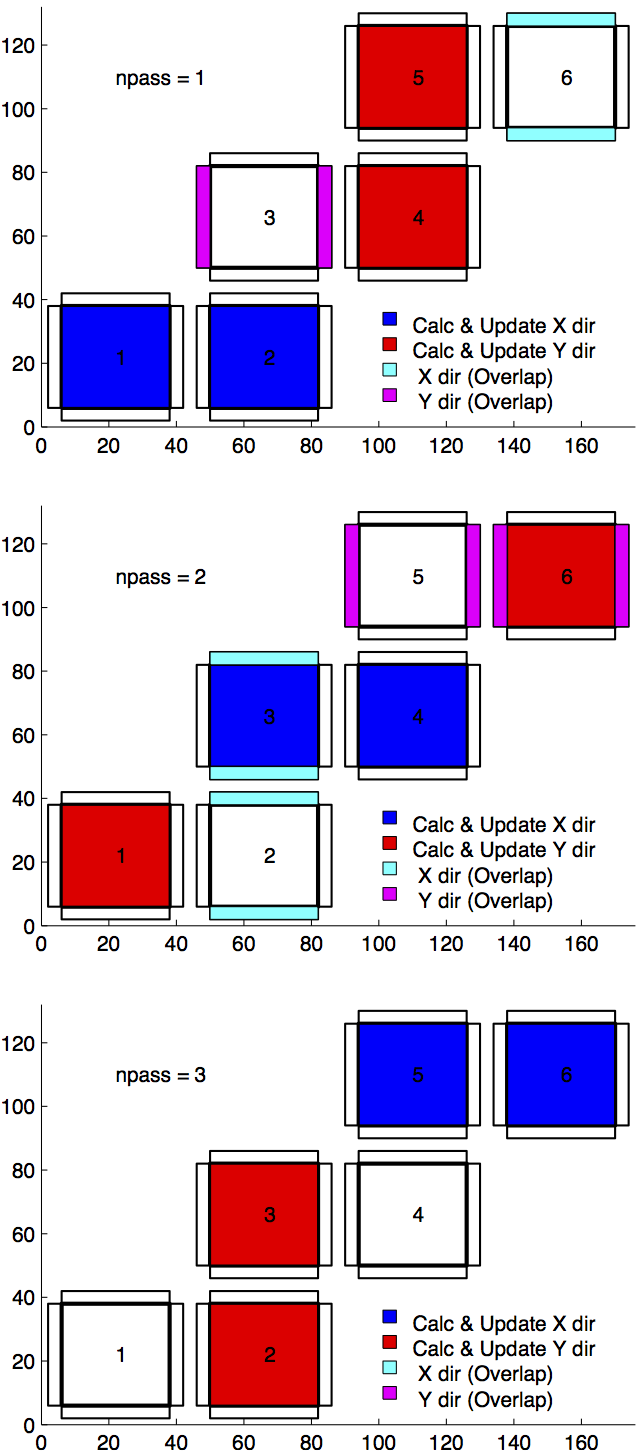Figure 2.11 Multi-dimensional advection time-stepping with cubed-sphere topology.

# 2.17.3. Comparison of advection schemes¶

Table 2.2 shows a summary of the different advection schemes available in MITgcm. “AB” stands for Adams-Bashforth and “DST” for direct space-time. The code corresponds to the number used to select the corresponding advection scheme in the parameter file (e.g., tempAdvScheme=3 in file data selects the 3rd order upwind advection scheme for temperature advection).

Code

Use AB?

Use multi-dim?

Stencil (1-D)

1st order upwind

1

no

yes*

3

linear $$\tau$$, non-linear $$\vec{v}$$

centered 2nd order

2

yes

no

3

linear

3rd order upwind

3

yes

no

5

linear $$\tau$$

centered 4th order

4

yes

no

5

linear

2nd order DST (Lax-Wendroff)

20

no

yes*

3

linear $$\tau$$, non-linear $$\vec{v}$$

3rd order DST

30

no

yes*

5

linear $$\tau$$, non-linear $$\vec{v}$$

2nd order flux limiters

77

no

yes*

5

non-linear

3rd order DST flux limiter

33

no

yes*

5

non-linear

piecewise parabolic w/“null” limiter

40

no

yes

7

non-linear

piecewise parabolic w/“mono” limiter

41

no

yes

7

non-linear

piecewise parabolic w/“weno” limiter

42

no

yes

7

non-linear

piecewise quartic w/“null” limiter

50

no

yes

9

non-linear

piecewise quartic w/“mono” limiter

51

no

yes

9

non-linear

piecewise quartic w/“weno” limiter

52

no

yes

9

non-linear

7th order one-step method w/monotonicity preserving limiter

7

no

yes

9

non-linear

second order-moment Prather

80

no

yes

3

non-linear

second order-moment Prather w/limiter

81

no

yes

3

non-linear

yes* indicates that either the multi-dim advection algorithm or standard approach can be utilized, controlled by a namelist parameter multiDimAdvection (in these cases, given that these schemes was designed to use multi-dim advection, using the standard approach is not recommended). The minimum size of the required tile overlap region (OLx, OLx) is (stencil size -1)/2. The minimum overlap required by the model in general is 2, so for some of the above choices the advection scheme will not cost anything in terms of an additional overlap requirement, but especially given a small tile size, using scheme 7 for example would require costly additional overlap points (note a cube sphere grid with a “wet-corner” requires doubling this overlap!) In the ‘Comments’ column, $$\tau$$ refers to tracer advection, $$\vec{v}$$ momentum advection.

Shown in Figure 2.12 and Figure 2.13 is a 1-D comparison of advection schemes. Here we advect both a smooth hill and a hill with a more abrupt shock. Figure 2.12 shown the result for a weak flow (low Courant number) whereas Figure 2.13 shows the result for a stronger flow (high Courant number).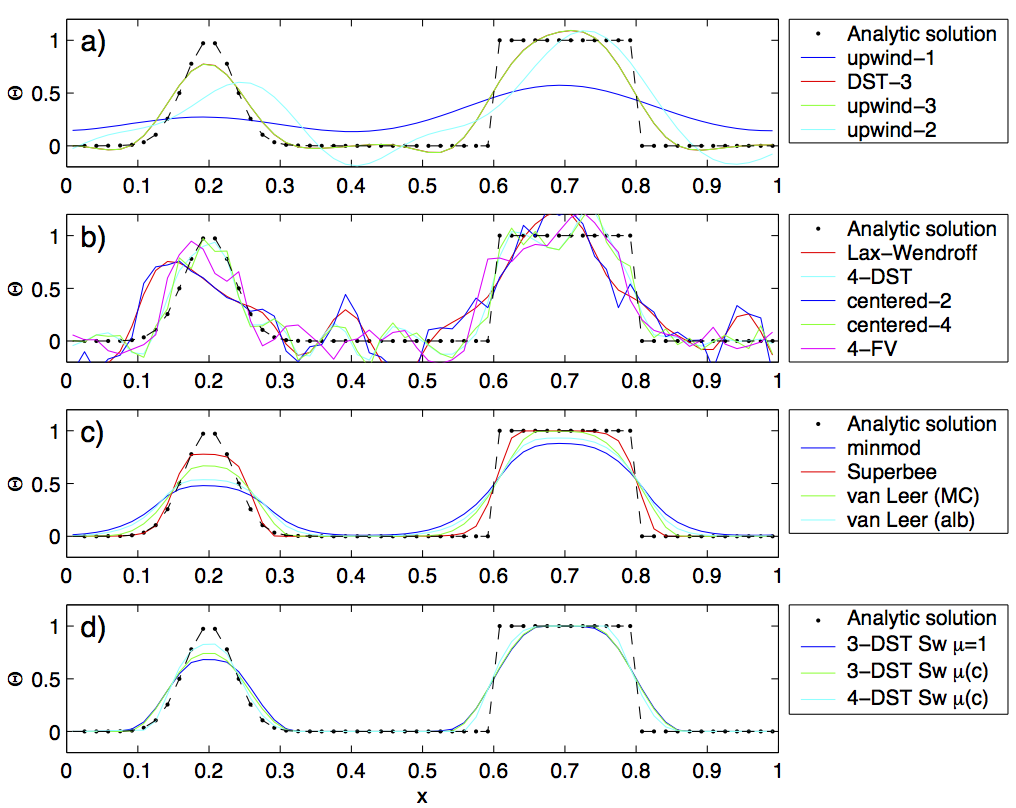Figure 2.12 Comparison of 1-D advection schemes: Courant number is 0.05 with 60 points and solutions are shown for T=1 (one complete period). a) Shows the upwind biased schemes; first order upwind, DST3, third order upwind and second order upwind. b) Shows the centered schemes; Lax-Wendroff, DST4, centered second order, centered fourth order and finite volume fourth order. c) Shows the second order flux limiters: minmod, Superbee, MC limiter and the van Leer limiter. d) Shows the DST3 method with flux limiters due to Sweby with $$\mu =1$$ , $$\mu =c/(1-c)$$ and a fourth order DST method with Sweby limiter, $$\mu =c/(1-c)$$ .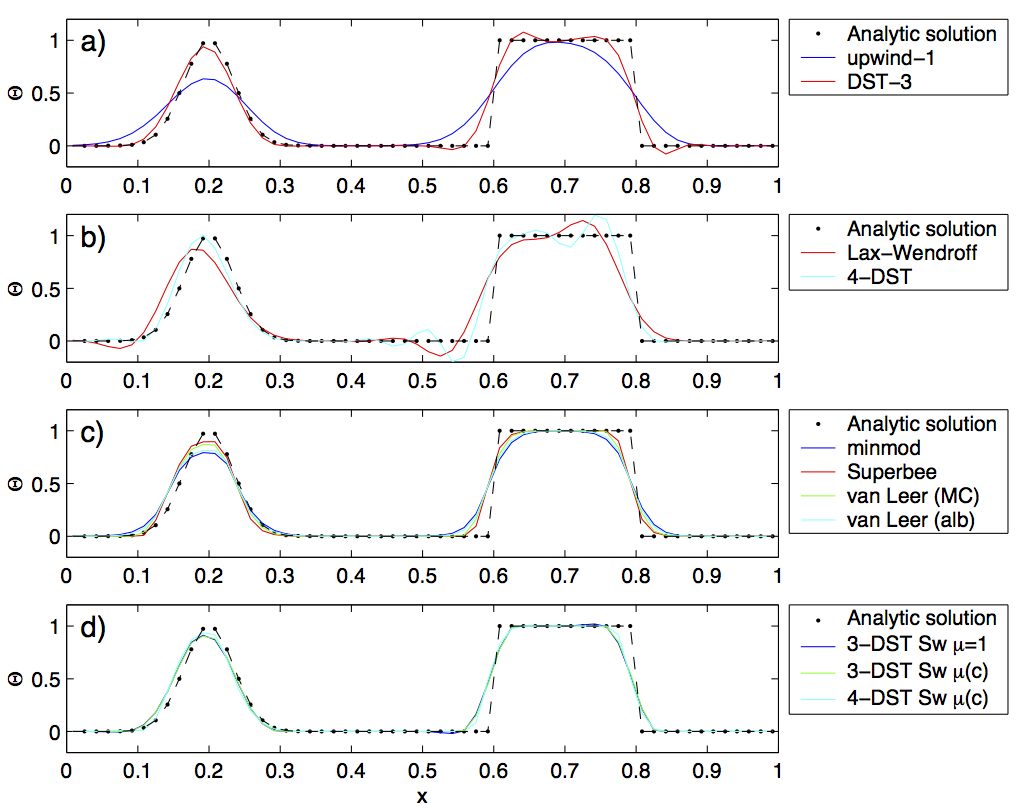Figure 2.13 Comparison of 1-D advection schemes: Courant number is 0.89 with 60 points and solutions are shown for T=1 (one complete period). a) Shows the upwind biased schemes; first order upwind and DST3. Third order upwind and second order upwind are unstable at this Courant number. b) Shows the centered schemes; Lax-Wendroff, DST4. Centered second order, centered fourth order and finite volume fourth order are unstable at this Courant number. c) Shows the second order flux limiters: minmod, Superbee, MC limiter and the van Leer limiter. d) Shows the DST3 method with flux limiters due to Sweby with $$\mu =1$$ , $$\mu =c/(1-c)$$ and a fourth order DST method with Sweby limiter, $$\mu =c/(1-c)$$ .

Figure 2.14, Figure 2.15 and Figure 2.16 show solutions to a simple diagonal advection problem using a selection of schemes for low, moderate and high Courant numbers, respectively. The top row shows the linear schemes, integrated with the Adams-Bashforth method. Theses schemes are clearly unstable for the high Courant number and weakly unstable for the moderate Courant number. The presence of false extrema is very apparent for all Courant numbers. The middle row shows solutions obtained with the unlimited but multi-dimensional schemes. These solutions also exhibit false extrema though the pattern now shows symmetry due to the multi-dimensional scheme. Also, the schemes are stable at high Courant number where the linear schemes weren’t. The bottom row (left and middle) shows the limited schemes and most obvious is the absence of false extrema. The accuracy and stability of the unlimited non-linear schemes is retained at high Courant number but at low Courant number the tendency is to lose amplitude in sharp peaks due to diffusion. The one dimensional tests shown in Figure 2.12 and Figure 2.13 show this phenomenon.

Finally, the bottom left and right panels use the same advection scheme but the right does not use the multi-dimensional method. At low Courant number this appears to not matter but for moderate Courant number severe distortion of the feature is apparent. Moreover, the stability of the multi-dimensional scheme is determined by the maximum Courant number applied of each dimension while the stability of the method of lines is determined by the sum. Hence, in the high Courant number plot, the scheme is unstable.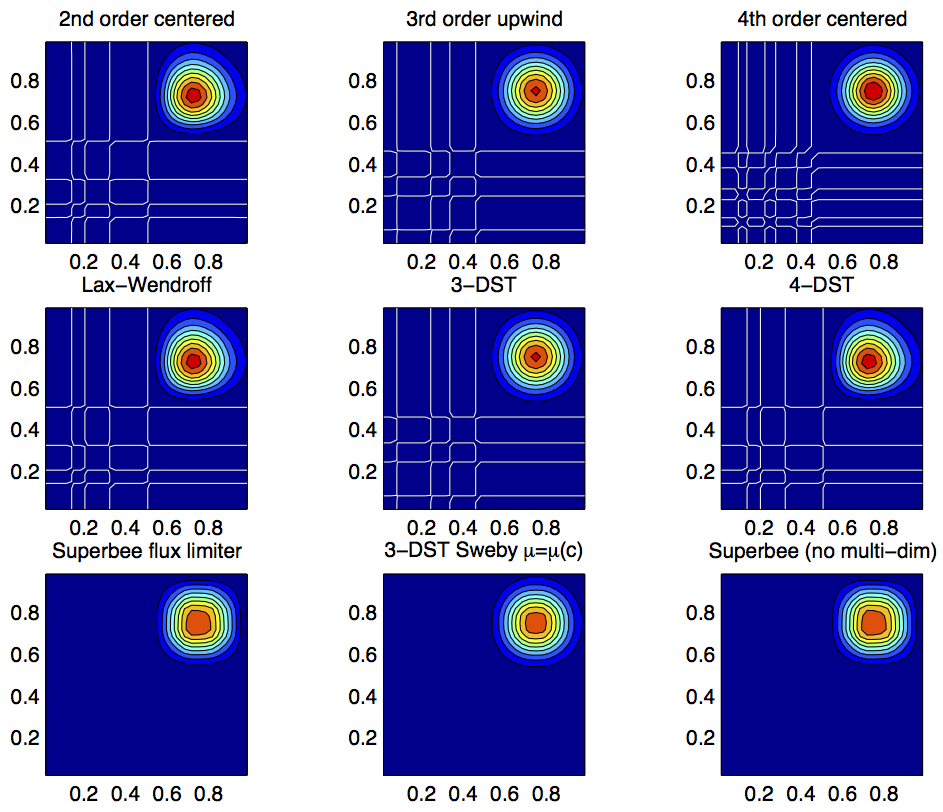Figure 2.14 Comparison of advection schemes in two dimensions; diagonal advection of a resolved Gaussian feature. Courant number is 0.01 with 30 $$\times$$ 30 points and solutions are shown for T=1/2. White lines indicate zero crossing (ie. the presence of false minima). The left column shows the second order schemes; top) centered second order with Adams-Bashforth, middle) Lax-Wendroff and bottom) Superbee flux limited. The middle column shows the third order schemes; top) upwind biased third order with Adams-Bashforth, middle) third order direct space-time method and bottom) the same with flux limiting. The top right panel shows the centered fourth order scheme with Adams-Bashforth and right middle panel shows a fourth order variant on the DST method. Bottom right panel shows the Superbee flux limiter (second order) applied independently in each direction (method of lines).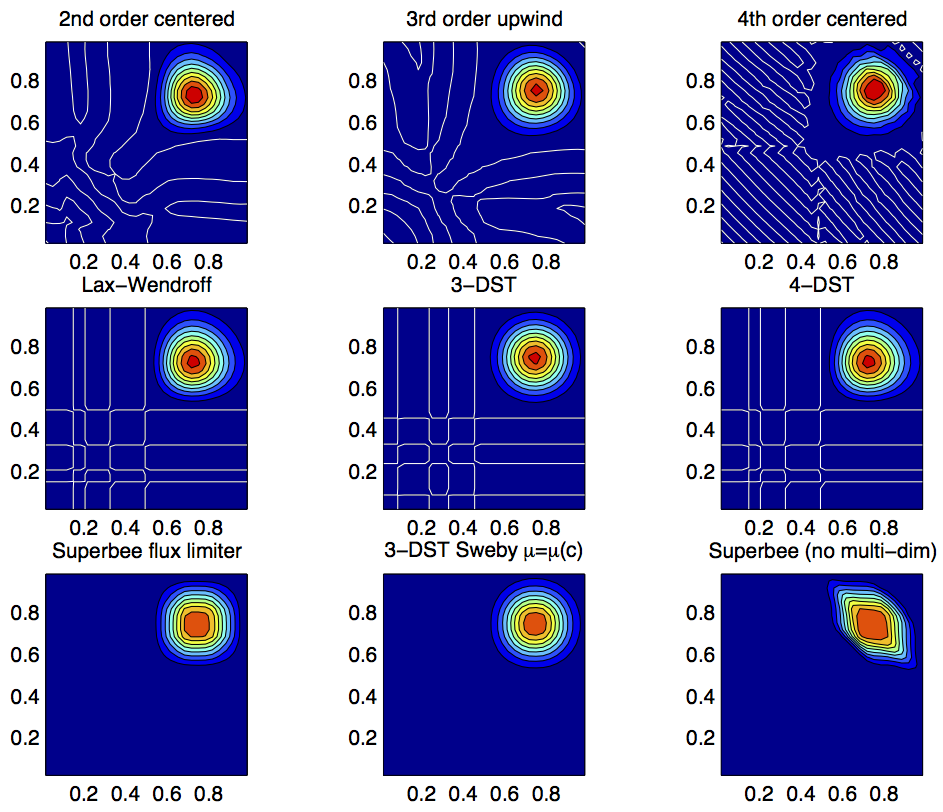Figure 2.15 Comparison of advection schemes in two dimensions; diagonal advection of a resolved Gaussian feature. Courant number is 0.27 with 30 $$\times$$ 30 points and solutions are shown for T=1/2. White lines indicate zero crossing (ie. the presence of false minima). The left column shows the second order schemes; top) centered second order with Adams-Bashforth, middle) Lax-Wendroff and bottom) Superbee flux limited. The middle column shows the third order schemes; top) upwind biased third order with Adams-Bashforth, middle) third order direct space-time method and bottom) the same with flux limiting. The top right panel shows the centered fourth order scheme with Adams-Bashforth and right middle panel shows a fourth order variant on the DST method. Bottom right panel shows the Superbee flux limiter (second order) applied independently in each direction (method of lines).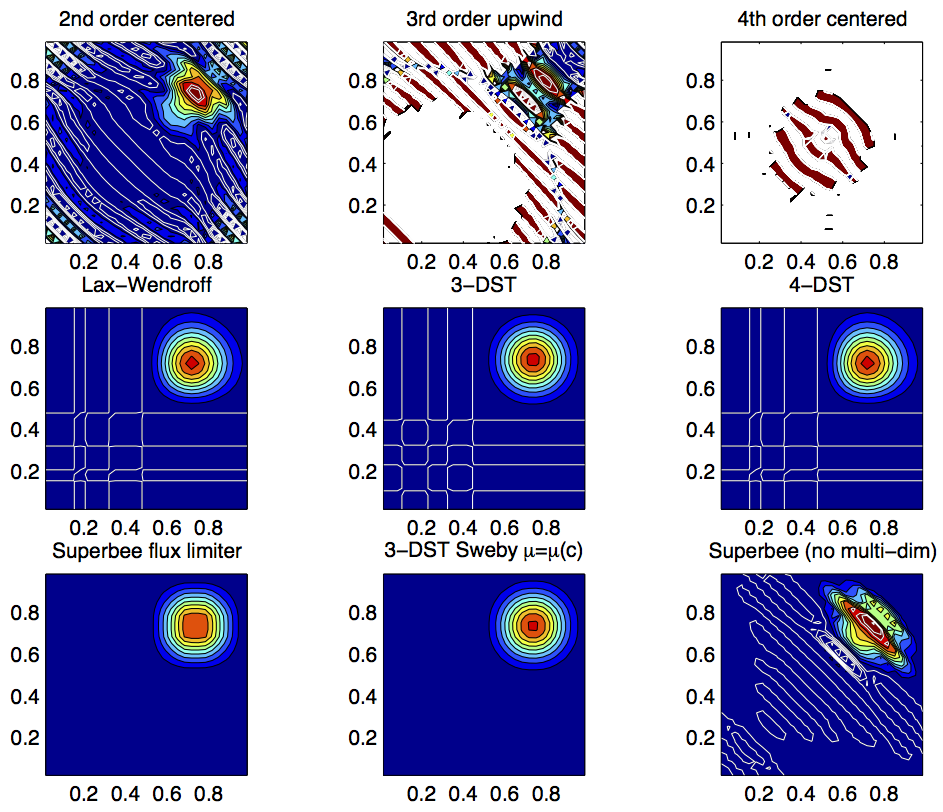Figure 2.16 Comparison of advection schemes in two dimensions; diagonal advection of a resolved Gaussian feature. Courant number is 0.47 with 30 $$\times$$ 30 points and solutions are shown for T=1/2. White lines indicate zero crossings and initial maximum values (ie. the presence of false extrema). The left column shows the second order schemes; top) centered second order with Adams-Bashforth, middle) Lax-Wendroff and bottom) Superbee flux limited. The middle column shows the third order schemes; top) upwind biased third order with Adams-Bashforth, middle) third order direct space-time method and bottom) the same with flux limiting. The top right panel shows the centered fourth order scheme with Adams-Bashforth and right middle panel shows a fourth order variant on the DST method. Bottom right panel shows the Superbee flux limiter (second order) applied independently in each direction (method of lines).

With many advection schemes implemented in the code two questions arise: “Which scheme is best?” and “Why don’t you just offer the best advection scheme?”. Unfortunately, no one advection scheme is “the best” for all particular applications and for new applications it is often a matter of trial to determine which is most suitable. Here are some guidelines but these are not the rule;

• If you have a coarsely resolved model, using a positive or upwind biased scheme will introduce significant diffusion to the solution and using a centered higher order scheme will introduce more noise. In this case, simplest may be best.

• If you have a high resolution model, using a higher order scheme will give a more accurate solution but scale-selective diffusion might need to be employed. The flux limited methods offer similar accuracy in this regime.

• If your solution has shocks or propagating fronts then a flux limited scheme is almost essential.

• If your time-step is limited by advection, the multi-dimensional non-linear schemes have the most stability (up to Courant number 1).

• If you need to know how much diffusion/dissipation has occurred you will have a lot of trouble figuring it out with a non-linear method.

• The presence of false extrema is non-physical and this alone is the strongest argument for using a positive scheme.